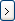# Using an Input Formula

An input formula lets you populate an edit box control with a string built up by values from variables linked to other controls on the data card.

To use an input formula, you create one or more list controls and an edit box control. The edit box control contains an input formula that is mapped to the variables of the list controls.

This example describes how to use an input formula to create a project name in a template card field.

To create the input formula:

1. Create a new data card.
2. For Card type, select Template Card.
3. Add three list controls and link them to variables. For example:
• City
• Region
• Code
4. Add an edit box to hold the combined project name to be created by the input formula.
5. In the Edit-box properties, under Input formula:
1. Type a string (for example, Prj-). This static text does not change when the formula is evaluated.
You can add static text at any point in the formula.
2. Clickto add the first list control variable (for example, City) to the formula.
3. Build up the rest of the formula by adding additional variables (for example, Region and Code).
To use a bigger input field, click Browse (...).
6. Save the card.
When a user completes the template card by selecting from the list controls, the project name is based on those selections because it is controlled by the input formula.

For example, if the user selects Stockholm from the City list, B from the Region list, and 112 from the Code list, the value of the Project Name edit box will be Prj-StockholmB112.